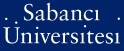# On lelong-bremermann lemma

Aytuna, Aydın and Zakharyuta, Vyacheslav (2008) On lelong-bremermann lemma. Proceedings of the American Mathematical Society, 136 (5). pp. 1733-1742. ISSN 1088-6826 (e) ISSN 0002-9939 (p)Preview
PDF - Requires a PDF viewer such as GSview, Xpdf or Adobe Acrobat Reader
193Kb

Official URL: http://dx.doi.org/10.1090/S0002-9939-08-09166-1

## Abstract

The main theorem of this note is the following refinement of the well-known Lelong-Bremermann Lemma: Let u be a continuous plurisubharmonic function on a Stein manifold. of dimension n. Then there exists an integer m <= 2n + 1, natural numbers p(s), and analytic mappings G(s) = (g(j)((s))): Omega -> C-m, s = 1, 2,..., such that the sequence of functions u(s) (z) = 1/p(s) max (ln vertical bar g(j)((s)) (z)vertical bar : j = 1,..., m converges to u uniformly on each compact subset of Omega. In the case when Omega is a domain in the complex plane, it is shown that one can take m = 2 in the theorem above (Section 3); on the other hand, for n-circular plurisubharmonic functions in C-n the statement of this theorem is true with m = n + 1 (Section 4). The last section contains some remarks and open questions.

Item Type: Article Plurisubharmonic functions, Lelong-Bremermann Lemma Q Science > QA Mathematics > QA299.6-433 Analysis 10998 Vyacheslav Zakharyuta 16 Dec 2008 11:11 16 Dec 2008 15:01

### Available Versions of this Item

Repository Staff Only: item control page